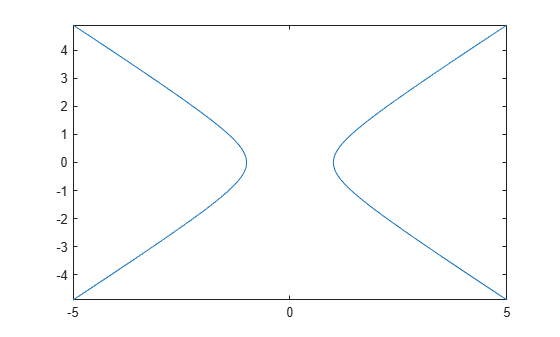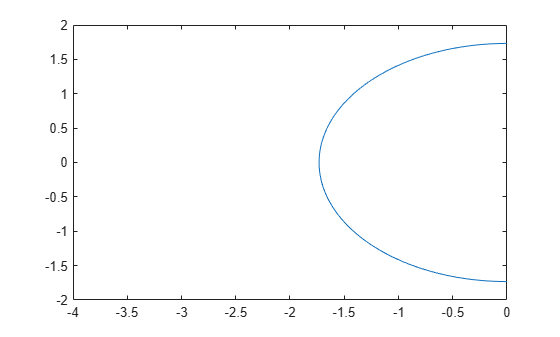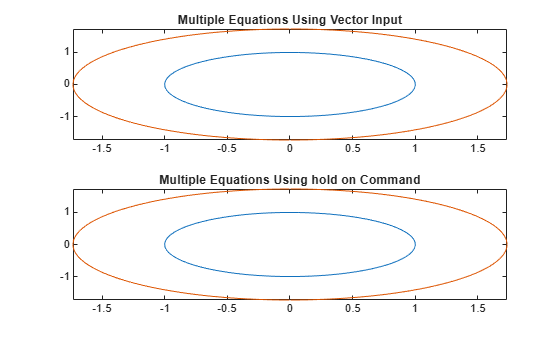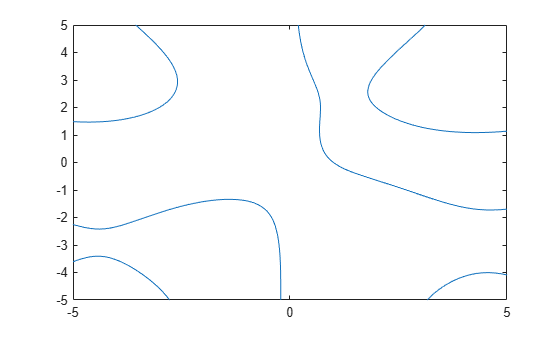# fimplicit

Plot implicit symbolic equation or function

## Syntax

fimplicit(f)
fimplicit(f,[min max])
fimplicit(f,[xmin xmax ymin ymax])
fimplicit(___,LineSpec)
fimplicit(___,Name,Value)
fimplicit(ax,___)
fi = fimplicit(___)

## Description

example

fimplicit(f) plots the implicit symbolic equation or function f over the default interval [-5 5] for x and y.
fimplicit(f,[min max]) plots f over the interval min < x < max and min < y < max.

example

fimplicit(f,[xmin xmax ymin ymax]) plots f over the interval xmin < x < xmax and ymin < y < ymax. The fimplicit function uses symvar to order the variables and assign intervals.

example

fimplicit(___,LineSpec) uses LineSpec to set the line style, marker symbol, and line color.

example

fimplicit(___,Name,Value) specifies line properties using one or more Name,Value pair arguments. Use this option with any of the input argument combinations in the previous syntaxes. Name,Value pair settings apply to all the lines plotted. To set options for individual lines, use the objects returned by fimplicit.
fimplicit(ax,___) plots into the axes specified by ax instead of the current axes gca.

example

fi = fimplicit(___) returns an implicit function line object. Use the object to query and modify properties of a specific line. For details, see ImplicitFunctionLine Properties.

## Examples

collapse all

Plot the hyperbola ${x}^{2}-{y}^{2}=1$ by using fimplicit. The fimplicit function uses the default interval of $\left[-5,5\right]$ for $x$ and $y$.

syms x y fimplicit(x^2 - y^2 == 1)Plot the hyperbola described by the function $f\left(x,y\right)={x}^{2}-{y}^{2}-1$ by first declaring the symbolic function f(x,y) using syms. The fimplicit function uses the default interval of $\left[-5,5\right]$ for $x$ and $y$.

syms f(x,y) f(x,y) = x^2 - y^2 - 1; fimplicit(f)Plot half of the circle ${x}^{2}+{y}^{2}=3$ by using the intervals $-4 and $-2. Specify the plotting interval as the second argument of fimplicit.

syms x y circle = x^2 + y^2 == 3; fimplicit(circle, [-4 0 -2 2])You can plot multiple equations either by passing the inputs as a vector or by using hold on to successively plot on the same figure. If you specify LineSpec and Name-Value arguments, they apply to all lines. To set options for individual plots, use the function handles returned by fimplicit.

Divide a figure into two subplots by using subplot. On the first subplot, plot ${x}^{2}+{y}^{2}==1$ and ${x}^{2}+{y}^{2}==3$ using vector input. On the second subplot, plot the same inputs by using hold on.

syms x y circle1 = x^2 + y^2 == 1; circle2 = x^2 + y^2 == 3; subplot(2,1,1) fimplicit([circle1 circle2]) title('Multiple Equations Using Vector Input') subplot(2,1,2) fimplicit(circle1) hold on fimplicit(circle2) title('Multiple Equations Using hold on Command') hold offPlot three concentric circles of increasing diameter. For the first line, use a linewidth of 2. For the second, specify a dashed red line style with circle markers. For the third, specify a cyan, dash-dot line style with asterisk markers. Display the legend.

syms x y circle = x^2 + y^2; fimplicit(circle == 1, 'Linewidth', 2) hold on fimplicit(circle == 2, '--or') fimplicit(circle == 3, '-.*c') legend('show','Location','best') hold offPlot $y\mathrm{sin}\left(x\right)+x\mathrm{cos}\left(y\right)=1$. Specify an output to make fimplicit return the plot object.

syms x y eqn = y*sin(x) + x*cos(y) == 1; fi = fimplicit(eqn)fi = ImplicitFunctionLine with properties: Function: [1x1 sym] Color: [0 0.4470 0.7410] LineStyle: '-' LineWidth: 0.5000 Show all properties 

Change the plotted equation to $x\mathrm{cos}\left(y\right)+y\mathrm{sin}\left(x\right)=0$ by using dot notation to set properties. Similarly, change the line color to red and line style to a dash-dot line. The horizontal and vertical lines in the output are artifacts that should be ignored.

fi.Function = x/cos(y) + y/sin(x) == 0; fi.Color = 'r'; fi.LineStyle = '-.';Plot $x\mathrm{cos}\left(y\right)+y\mathrm{sin}\left(x\right)=1$ over the interval $-2\pi and $-2\pi . Add a title and axis labels. Create the x-axis ticks by spanning the x-axis limits at intervals of pi/2. Display these ticks by using the XTick property. Create x-axis labels by using arrayfun to apply texlabel to S. Display these labels by using the XTickLabel property. Repeat these steps for the y-axis.

To use LaTeX in plots, see latex.

syms x y eqn = x*cos(y) + y*sin(x) == 1; fimplicit(eqn, [-2*pi 2*pi]) grid on title('x cos(y) + y sin(x) for -2\pi < x < 2\pi and -2\pi < y < 2\pi') xlabel('x') ylabel('y') ax = gca; S = sym(ax.XLim(1):pi/2:ax.XLim(2)); ax.XTick = double(S); ax.XTickLabel = arrayfun(@texlabel, S, 'UniformOutput', false); S = sym(ax.YLim(1):pi/2:ax.YLim(2)); ax.YTick = double(S); ax.YTickLabel = arrayfun(@texlabel, S, 'UniformOutput', false);When you zoom into a plot, fimplicit re-evaluates the plot automatically. This re-evaluation on zoom can reveal hidden detail at smaller scales.

Divide a figure into two by using subplot. Plot $x\mathrm{cos}\left(y\right)+y\mathrm{sin}\left(1/x\right)=0$ in both the first and second subplots. Zoom into the second subplot by using zoom. The zoomed subplot shows detail that is not visible in the first subplot.

syms x y eqn = x*cos(y) + y*sin(1/x) == 0; subplot(2,1,1) fimplicit(eqn) subplot(2,1,2) fimplicit(eqn) zoom(2)## Input Arguments

collapse all

Implicit equation or function to plot, specified as a symbolic equation, expression, or function. If the right-hand side is not specified, then it is assumed to be 0.

Plotting range for x and y, specified as a vector of two numbers. The default range is [-5 5].

Plotting range for x and y, specified as a vector of four numbers. The default range is [-5 5 -5 5].

Axes object. If you do not specify an axes object, then fimplicit uses the current axes gca.

Line style, marker, and color, specified as a character vector or string containing symbols. The symbols can appear in any order. You do not need to specify all three characteristics (line style, marker, and color). For example, if you omit the line style and specify the marker, then the plot shows only the marker and no line.

Example: '--or' is a red dashed line with circle markers

Line StyleDescription
-Solid line
--Dashed line
:Dotted line
-.Dash-dot line
MarkerDescription
'o'Circle
'+'Plus sign
'*'Asterisk
'.'Point
'x'Cross
'_'Horizontal line
'|'Vertical line
's'Square
'd'Diamond
'^'Upward-pointing triangle
'v'Downward-pointing triangle
'>'Right-pointing triangle
'<'Left-pointing triangle
'p'Pentagram
'h'Hexagram
ColorDescription

y

yellow

m

magenta

c

cyan

r

red

g

green

b

blue

w

white

k

black

### Name-Value Pair Arguments

Specify optional comma-separated pairs of Name,Value arguments. Name is the argument name and Value is the corresponding value. Name must appear inside quotes. You can specify several name and value pair arguments in any order as Name1,Value1,...,NameN,ValueN.

Example: 'Marker','o','MarkerFaceColor','red'

The function line properties listed here are only a subset. For a complete list, see ImplicitFunctionLine Properties.

Number of evaluation points per direction, specified as a number. The default is 151.

Line color, specified as an RGB triplet, a hexadecimal color code, a color name, or a short name.

For a custom color, specify an RGB triplet or a hexadecimal color code.

• An RGB triplet is a three-element row vector whose elements specify the intensities of the red, green, and blue components of the color. The intensities must be in the range [0,1]; for example, [0.4 0.6 0.7].

• A hexadecimal color code is a character vector or a string scalar that starts with a hash symbol (#) followed by three or six hexadecimal digits, which can range from 0 to F. The values are not case sensitive. Thus, the color codes '#FF8800', '#ff8800', '#F80', and '#f80' are equivalent.

Alternatively, you can specify some common colors by name. This table lists the named color options, the equivalent RGB triplets, and hexadecimal color codes.

Color NameShort NameRGB TripletHexadecimal Color CodeAppearance
'red''r'[1 0 0]'#FF0000''green''g'[0 1 0]'#00FF00''blue''b'[0 0 1]'#0000FF''cyan' 'c'[0 1 1]'#00FFFF''magenta''m'[1 0 1]'#FF00FF''yellow''y'[1 1 0]'#FFFF00''black''k'[0 0 0]'#000000''white''w'[1 1 1]'#FFFFFF'Here are the RGB triplets and hexadecimal color codes for the default colors MATLAB® uses in many types of plots.

[0 0.4470 0.7410]'#0072BD'[0.8500 0.3250 0.0980]'#D95319'[0.9290 0.6940 0.1250]'#EDB120'[0.4940 0.1840 0.5560]'#7E2F8E'[0.4660 0.6740 0.1880]'#77AC30'[0.3010 0.7450 0.9330]'#4DBEEE'[0.6350 0.0780 0.1840]'#A2142F'Example: 'blue'

Example: [0 0 1]

Example: '#0000FF'

Line style, specified as one of the options listed in this table.

Line StyleDescriptionResulting Line
'-'Solid line'--'Dashed line':'Dotted line'-.'Dash-dotted line'none'No lineNo line

Line width, specified as a positive value in points, where 1 point = 1/72 of an inch. If the line has markers, then the line width also affects the marker edges.

The line width cannot be thinner than the width of a pixel. If you set the line width to a value that is less than the width of a pixel on your system, the line displays as one pixel wide.

Marker symbol, specified as one of the values listed in this table. By default, the object does not display markers. Specifying a marker symbol adds markers at each data point or vertex.

ValueDescription
'o'Circle
'+'Plus sign
'*'Asterisk
'.'Point
'x'Cross
'_'Horizontal line
'|'Vertical line
'square' or 's'Square
'diamond' or 'd'Diamond
'^'Upward-pointing triangle
'v'Downward-pointing triangle
'>'Right-pointing triangle
'<'Left-pointing triangle
'pentagram' or 'p'Five-pointed star (pentagram)
'hexagram' or 'h'Six-pointed star (hexagram)
'none'No markers

Marker outline color, specified as 'auto', an RGB triplet, a hexadecimal color code, a color name, or a short name. The default value of 'auto' uses the same color as the Color property.

For a custom color, specify an RGB triplet or a hexadecimal color code.

• An RGB triplet is a three-element row vector whose elements specify the intensities of the red, green, and blue components of the color. The intensities must be in the range [0,1]; for example, [0.4 0.6 0.7].

• A hexadecimal color code is a character vector or a string scalar that starts with a hash symbol (#) followed by three or six hexadecimal digits, which can range from 0 to F. The values are not case sensitive. Thus, the color codes '#FF8800', '#ff8800', '#F80', and '#f80' are equivalent.

Alternatively, you can specify some common colors by name. This table lists the named color options, the equivalent RGB triplets, and hexadecimal color codes.

Color NameShort NameRGB TripletHexadecimal Color CodeAppearance
'red''r'[1 0 0]'#FF0000''green''g'[0 1 0]'#00FF00''blue''b'[0 0 1]'#0000FF''cyan' 'c'[0 1 1]'#00FFFF''magenta''m'[1 0 1]'#FF00FF''yellow''y'[1 1 0]'#FFFF00''black''k'[0 0 0]'#000000''white''w'[1 1 1]'#FFFFFF''none'Not applicableNot applicableNot applicableNo color

Here are the RGB triplets and hexadecimal color codes for the default colors MATLAB uses in many types of plots.

[0 0.4470 0.7410]'#0072BD'[0.8500 0.3250 0.0980]'#D95319'[0.9290 0.6940 0.1250]'#EDB120'[0.4940 0.1840 0.5560]'#7E2F8E'[0.4660 0.6740 0.1880]'#77AC30'[0.3010 0.7450 0.9330]'#4DBEEE'[0.6350 0.0780 0.1840]'#A2142F'Marker fill color, specified as 'auto', an RGB triplet, a hexadecimal color code, a color name, or a short name. The 'auto' value uses the same color as the MarkerEdgeColor property.

For a custom color, specify an RGB triplet or a hexadecimal color code.

• An RGB triplet is a three-element row vector whose elements specify the intensities of the red, green, and blue components of the color. The intensities must be in the range [0,1]; for example, [0.4 0.6 0.7].

• A hexadecimal color code is a character vector or a string scalar that starts with a hash symbol (#) followed by three or six hexadecimal digits, which can range from 0 to F. The values are not case sensitive. Thus, the color codes '#FF8800', '#ff8800', '#F80', and '#f80' are equivalent.

Alternatively, you can specify some common colors by name. This table lists the named color options, the equivalent RGB triplets, and hexadecimal color codes.

Color NameShort NameRGB TripletHexadecimal Color CodeAppearance
'red''r'[1 0 0]'#FF0000''green''g'[0 1 0]'#00FF00''blue''b'[0 0 1]'#0000FF''cyan' 'c'[0 1 1]'#00FFFF''magenta''m'[1 0 1]'#FF00FF''yellow''y'[1 1 0]'#FFFF00''black''k'[0 0 0]'#000000''white''w'[1 1 1]'#FFFFFF''none'Not applicableNot applicableNot applicableNo color

Here are the RGB triplets and hexadecimal color codes for the default colors MATLAB uses in many types of plots.

[0 0.4470 0.7410]'#0072BD'[0.8500 0.3250 0.0980]'#D95319'[0.9290 0.6940 0.1250]'#EDB120'[0.4940 0.1840 0.5560]'#7E2F8E'[0.4660 0.6740 0.1880]'#77AC30'[0.3010 0.7450 0.9330]'#4DBEEE'[0.6350 0.0780 0.1840]'#A2142F'Example: [0.3 0.2 0.1]

Example: 'green'

Example: '#D2F9A7'

Marker size, specified as a positive value in points, where 1 point = 1/72 of an inch.

## Output Arguments

collapse all

One or more implicit function line objects, returned as a scalar or a vector. You can use these objects to query and modify properties of a specific line. For a list of properties, see ImplicitFunctionLine Properties.

## Algorithms

fimplicit assigns the symbolic variables in f to the x axis, then the y axis, and symvar determines the order of the variables to be assigned. Therefore, variable and axis names might not correspond. To force fimplicit to assign x or y to its corresponding axis, create the symbolic function to plot, then pass the symbolic function to fimplicit.

For example, the following code plots the roots of the implicit function f(x,y) = sin(y) in two ways. The first way forces the waves to oscillate with respect to the y axis. The second way assigns y to the x axis because it is the first (and only) variable in the symbolic function.

syms x y; f(x,y) = sin(y); intvl = [-6 6]*pi; figure; subplot(2,1,1) fimplicit(f,intvl); subplot(2,1,2) fimplicit(f(x,y),intvl); % Or fimplicit(sin(y) == 0,intvl);### Topics

Introduced in R2016b

## Support

#### Mathematical Modeling with Symbolic Math Toolbox

Get examples and videos